In physics, power is the rate at which energy conversions take place. Science and technology education from FT Exploring.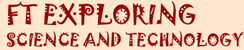If this page is helpful, please recommend: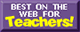Energy Pages: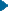Energy Introduction PageEnergy Changes HappenHow Many Types of Energy?Heat Flows Through ItFirst Law of ThermodynamicsFirst Law of Thermodynamics P2NEW: Wind Turbine EfficiencyEnergy is EverythingEnergy Changes in Bows, Catapults, and FleasEnergy Flows Through LifeThe Great Energy PyramidsResearching for Mr. EnergySecond Law of ThermodynamicsPhotosynthesis Intro PageEnergy Flows Through Life

Other FT Exploring PagesDr GalapagosWhat is an Engineer, ReallySite MapLink PagesLesson PlansContact UsPower - How Fast is Your Energy Being Converted?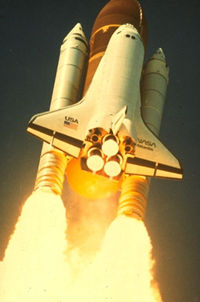The space shuttle's power at takeoff is about 11,000,000,000 Watts, or 11 Giga Watts (GW), or 11,000,000,000 Joules of energy being converted from stored chemical energy to kinetic energy and thermal energy every second. Eleven Giga Watts is the same as 14.75 million horsepower, or 10,424,048 BTU's of chemical energy converting to kinetic energy each second.Confused about the difference between power and energy? I don't mean the kind of power politicians crave. I'm talking about the kind of power related to energy that you learn about in physics.

In physics, power and energy do go hand in hand, but they're not the same thing. Whenever energy is changing or moving, there also is power, telling us how fast the energy is being transformed.

Energy, as described in other pages on this site, is the property of matter that makes things happen. To make things happen, energy must change form or change locations. Power is a measure of how fast those energy changes are happening.

Energy comes in set quantities like Joules (1 Newton-meter), foot-pounds, Calories, or BTU's (British Thermal Units).

Power is a rate. In physics, rates describe how something changes with time.

For example, velocity is the well known rate of distance traveled per length of time. If we measured Superman running 5000 kilometers (km) in 5 seconds of time, we could say Superman's velocity was 5000 kilometers (km) per 5 seconds (s).
Then you might say, "Well Wonder Woman just ran 6400 km in 7 seconds". Who's faster?

To make it more consistent for comparisons, we like to keep our time units as 1, so we could divide 5000 km by 5 seconds. Now we can say Superman runs "1000 kilometers per 1 second" (5000 km/5 s), or just 1000 km/s. Wonder Woman only runs 914.3 km/s (6400/7). Maybe she was just jogging.

Power is the rate of energy change per length of time. It tells us the quantity of energy that changed during a certain period of time. So the units of power are chunks of energy per amount of time, like 1 Joule per second (which is the same as 1 Watt), or 1000 Btu per hour, or 550 foot-pounds of energy per second (which is 1 horsepower).

Light Bulbs, Heaters, & Watts:
As we just said above, the unit of power called a Watt is the measure of energy being converted at the rate of 1 Joule every second. A 50 Watt light bulb converts 50 Joules of electrical energy to thermal energy (heat) and electromagnetic radiation (light) every second.
A 100 Watt light bulb converts 100 Joules every second, mostly to heat and a little light also. Yes, it's true, a typical incandescent bulb is mostly making heat. That's why it's so hot to touch. Only about 2 percent of the electical energy being converted in a light bulb becomes light. The other 98% is making heat that warms up the air in your house. Not so bad in the winter, but definitely unwanted on a hot summer day.

 Units of Energy & Power An important and useful measure of energy is the Joule (J). A Joule is officially the amount of energy required to exert a force of 1 Newton through a distance of 1 meter. So one Joule equals 1 Newton-meter or 1 N-m (1 J = 1 N-m). That's the mechanical definition. Thermal energy can also be defined, as we show a couple of paragraphs below. A Joule is not a lot of energy, unless you're a hummingbird. A hummingbird in flight converts 1 Joule of energy to heat, kinetic energy, and aerodynamic lift, every second. That means the little bird requires about 1 Watt (1 J/s) of power to fly. Energy units like Btu's and calories are generally defined in terms of thermal energy. A Btu is approximately the amount of energy needed to increase the thermal energy of 1 pound of water enough to raise it's temperature by 1 degree Farenheit. A calorie is the amount of energy needed to raise the temperature of 1 gram of water by 1 degree celsius. The Calories that dieters worry about, are really kilocalories (kcal) or 1000 calories. In other words the amount of energy required to increase the temperature of 1 kilogram (1000 grams) of water by 1 degree C. One Btu is equivalent to about 1055 Joules. The kilocalories that weight watchers count are equal to 4,186 Joules.How about that small electric heater in your family room. It sucks up 1500 Watts of power on the highest setting. That means it is converting 1500 Joules of electric energy directly into thermal energy every second. Pretty much the same thing that fifteen 100-Watt incandescent bulbs are doing.

Lifting Weights:
On earth it takes about 10 Newton-meters (N-m) of energy to raise a 1 kilogram mass to a height of 1 meter. Since 1 N-m equals 1 Joule, that's 10 Joules. If it takes 1 second to lift the weight 1 meter, than you have converted 10 Joules of energy to potential energy in one second. That's 10 Watts of power. If you lift a 2 kg weight 1 meter in 1 second, then the rate of energy conversion is 2 x 10 = 20 Joules per second, or 20 Watts of power.

If you can keep lifting a 1 kg weight to a height of 1 meter every second, weight after weight after weight, then you will be steadily producing 10 Watts of power. Your arm would get tired after a while.

Let's slow down. Suppose you move the 1 kilogram weight more slowly so that it takes 2 seconds to lift it 1 meter. Now the rate of work is 10 Joules (Newton-meters) of energy every 2 seconds. Ten Joules divided by 2 seconds is 5 Joules per second, or 5 Watts. Remember that 1 Watt is equal to 1 joule of energy conversion every second.

Power in a Locomotive
On a more dramatic scale, a locomotive is a device designed to convert the chemical potential energy stored in diesel fuel into the kinetic energy of thousands of tons of freight, and itself, moving down the track.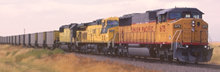Modern locomotives in the US range from about 4000 to 6000 horsepower. One horsepower is equal to about 746 Joules of energy conversion per second ( 746 Watts).A typical gallon of diesel fuel stores about 130,000 Btu's (more than 137 million Joules) of chemical energy.

If the diesel engine of a 6000 horsepower (4476 kilowatts) locomotive has a thermal efficiency of 42% (pretty good), then it will burn about 280 gallons of fuel every hour. Almost 30 million Btu's of stored chemical energy are being converted into thermal energy and kinetic energy every hour.
( As the 2nd law of themodynamics tells us, each of those little concentrated gallons of useful energy are now lost to us forever. The First Law tells us the energy is still around, but after being turned into useful kinetic energy of the moving train, the energy, all of the energy, through friction, becomes low-grade thermal energy that simply can't be reused. The useful high-grade energy, formerly stored in the fuel, is gone and we'll never get it back.)

Human PowerHow much power do we humans generate going about our daily activities?
Let's take a man about my size. I weigh about 75 kg (165 pounds) of solid muscle. Okay, stop laughing, there's some fat in there. Anyway someone like me, just sitting around (a good way to add more fat) reading or watching TV (a stupid waste of time compared to reading, if you're determined to add fat), converts about 68 Calories of food energy into heat and bodily functions (pumping blood, breathing, cellular processes, etc.) every hour. That's equal to about 79 Watts of power, just to sit and stay alive. Me and the 75 Watt bulb are both heating up the room at about the same rate (but the light bulb is brightening things up a bit).

If I get off my duff and start walking briskly, say 3.5 miles per hour, then I am converting about 330 Calories of food energy to motion and thermal energy every hour. That's about 383 Watts of power. Pretty good, huh? Watch the fat melting away. Keep walking every day, and it really will.

Now, if I really get motivated and start jogging at a pretty good clip, but not quite a full out sprint, I might manage to convert 642 Calories of energy into motion and heat every hour (though it is doubtful I could keep up that pace for an hour). Now I am generating 745 Watts of power, or 745 Newton-meters (Joules) of energy every second. That's almost 1 horsepower! Forget about it. I can't do that.

Say It Again
Power is the rate at which energy is converted. Whenever energy changes form, or moves from place to place (heat flow), the rate at which it makes those changes is described as power. If a device converts one Joule of energy every second the power is 1 Watt. If a device converts one Joule of energy every 10 seconds, the power is one tenth of a Watt (1 J/10 seconds = 0.1 Watts). If a device converts 1000 Joules of energy every second, the power is 1000 Joules per second, or 1000 Watts (1 kilowatt).

The Watt is a unit of power that is equal to one Joule of energy being converted every second. Power is always some unit of energy (like Joules, foot-pounds, Btu's, calories) divided by some unit of time (like seconds, minutes, hours, or months). One Btu per month is a very small unit of power.

Back to the Energy Intro Page

If this page was helpful, please recommend:If this page is helpful, please recommend it:Visit my porch Study and support excellence. This is fun! Great music. Great lyrics! The best band you probably haven't heard of.At Latal music available here - free even (but generosity is good for your karma)©Copyright 2005, 2014 David Watson. All rights reserved. Everything in the FT Exploring web site is written and copyrighted, either by David Watson, or by someone else. For information concerning use of this material, click on the word Copyright to go to our legal page.